## Polar Plot

Based on the homogeneous sorting of objects against these 114 repositories we will get the number 57 which is distributed over the pair of objects whose difference is twenty-nine (29). So everything will happen to repeat in this 29.

(114/2)! = 57! = 1653 » 1653 / 57 = 29

``````--------+
| ⅓
+---   } ⅔
Case A | ⅓
+---------
| ⅓      |
-----------------+  Φ = ⅔
| ⅓      |
+---------
Case B | ⅓
+---   } ⅔
| ⅓
---------
``````

By this objects pairing there would exist a zone segregation numbers (28.29) on each of the pairs. Since 10 stands as the central zone then it would be a tensor between 29-10=19 and 28-10=18.

9 + 19 + 29 = 28 + 29 = (10+18) + (10+19) = 57

``````P7:(142857)

#  |  A   |  B   | ∑
------+------+------+-----
{1} |      |      |
------+      |      |
...  |  28  |  29  | 57
------+      |      |
{57} |      |      |
------+------+------+-----
58  |      |      |
------+      |      |
... |  29  |  28  | 57
------+      |      |
114  |      |      |
------+------+------+-----
|  57  |  57  | 114
``````

This zone is cenralized by the 20 objects while the tensor of 19 vs 18 is polarizing the 2x11x13=286 objects out of the pairing of 11 and 13 within the True Prime Pairs and finally be composed by 20/10 = 2 numbers of 285 and 286 that having a total of 571.

Φ((13,12),(12,18)) = Φ(13,((19,18),(18,43)) = Φ(13,37,61) = Φ(6,12,18)th prime

``````---+-----+-----+-----+-----+
1 |{19} | 1   |{20} | 21  |-----------------------
---+-----+-----+-----+-----+                       |
2 | 18  | 21  | 39  | 60  |-----------------      |
---+-----+-----+-----+-----+                 |     |
3 | 63  | 40  | 103 |{143}|-----------      |     |
---+-----+-----+-----+-----+           |     |     |
4 | 37  | 104 | 141 | 245 |-----      |     |     |
---+-----+-----+-----+-----+     |     |     |     |
5 | 10  | 142 | 152 | 294 |-{11}|{13} |{12} |{12} |{18}
---+-----+-----+-----+-----+     |     |     |     |
6 | 24  | 153 |{177}| 332 |-----      |     |     |
---+-----+-----+-----+-----+           |     |     |
7 | 75  | 178 | 253 | 431 |-----------      |     |
---+-----+-----+-----+-----+                 |     |
8 | 30  | 254 | 284 | 538 |-----------------      |
---+-----+-----+-----+-----+                       |
9 | 1   | 285 | 286 |{571}|-----------------------
===+=====+=====+=====+=====+
45 |{277}|
---+-----+
``````

However by the prime numbers this behaviour is not happened by the number of 57 but on the 157 as the 19+18=37th prime. So on the pairing of 57 to 114 above then one of them is forced to behave as 157-57=100 which is exactly the square of 10.

157 is the 37th prime number, a balanced prime, because the arithmetic mean of those primes yields 157. The next prime is 163 and the previous prime is 151, with which 157 forms a prime triplet. (Wikipedia)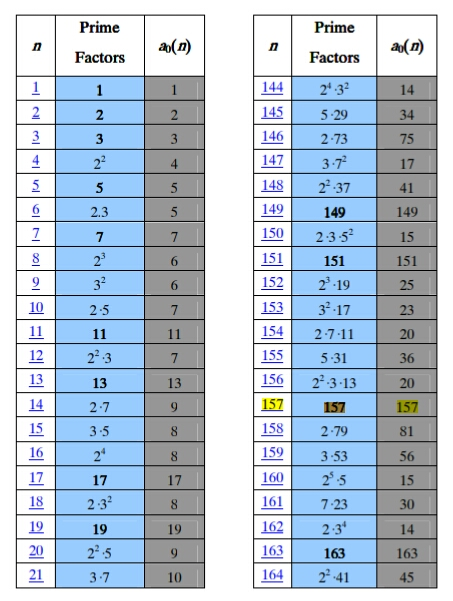By this square correlation between natural and prime numbers then the 571 would be separated by the 100 to 500 and 71 and finally by the form of (2,10) the 500 goes to 50 while 71 would be polarized to form 71x2=142 and 177 as shown on the table.

(10/2)π = 157 ⇄ (10^2)¹ + 11x7 = 177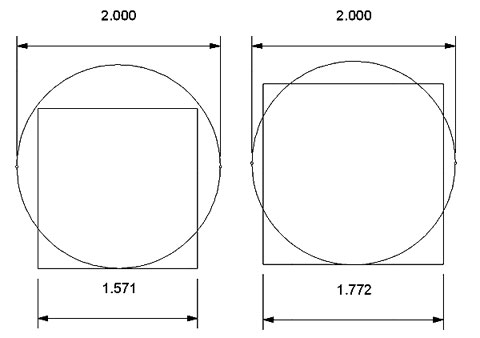That is why DNA is twisted and spinning in the scope of three (3) directions in three (3) dimensions which in its entirety resembles the shape of a trifoil. For XX genes this lead to 1600 objects but for XY genes there are different story.

When a 3 × 3 × 3 cube is made of 27 unit cubes, 26 of them are viewable as the exterior layer. (Wikipedia)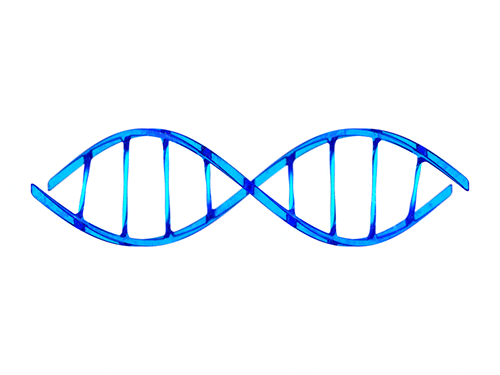From this arrangement we get the sum of all vectors with the sequence at number 247 where via the number one (1) makes 10 connected with 13 and 14 to the number twenty-seven (27).

Φ(11,13) = (114 - 10²) + 13 = 27 = 9 x 3 = 3 x 3 x 3 = 3 ^ 3

``````----------+----+----+----+----+----+----+----+----+----+----+----+----+----+----+
seq       | {1}|  2 |  3 |  4 |  5 | {6}| {7}|  8 |  9 | 10 | 11 | 12 | 13 | 14 |
----------+----+----+----+----+----+----+----+----+----+----+----+----+----+----+
num       | {3}| {4}|  3 |  4 |  5 |  2 |  3 |  2 |  2 |  1 |  2 |  5 |  1 |  1 |{38}
----------+----+----+----+----+----+----+----+----+----+----+----+----+----+----+---- } 285
seq x num |  3 |  8 |  9 | 16 | 25 |{12}|{21}| 16 | 18 | 10 | 22 | 60 |{13}|{14}|{247}
----------+----+----+----+----+----+----+----+----+----+----+----+----+----+----+
|-- 11 ---|              |-- 33 ---|                        |-- {27}--|
``````

Since there are no twin primes between 26² and 28² so they would goes to the rest object of 27. As you can see the formation of 69 objects from the number 29 ends in a 6 x 9 matrix centrally.

6 + (6x6) + 6 x (6+6) = 6 x (6+6) + 6 + (6x6) = 72 + 42 = 114

``````The Prime Recycling ζ(s):
(2,3), (29,89), (36,68), (72,42), (100,50), (2,3), (29,89), ...**infinity**
----------------------+-----+-----+-----+                                    ---
7 --------- 1,2:1|   1 |  30 |  40 | 71 (2,3) ‹-------------@----        |
|                +-----+-----+-----+-----+                        |      |
|  8 ‹------  3:2|   1 |  30 |  40 |  90 | 161 (7) ‹---           |      5¨  encapsulation
|  |             +-----+-----+-----+-----+             |          |      |
|  |  6 ‹-- 4,6:3|   1 |  30 | 200 | 231 (10,11,12) ‹--|---       |      |
|  |  |          +-----+-----+-----+-----+             |   |      |     ---
--|--|-----» 7:4|   1 |  30 |  40 | 200 | 271 (13) --›    | {5®} |      |
|  |          +-----+-----+-----+-----+                 |      |      |
--|---› 8,9:5|   1 |  30 | 200 | 231 (14,15) ---------›       |      7¨  abstraction
289        |          +-----+-----+-----+-----+-----+                  |      |
|          ----› 10:6|  20 |   5 |  10 |  70 |  90 | 195 (19) --› Φ   | {6®} |
--------------------+-----+-----+-----+-----+-----+                  |     ---
67 --------› 11:7|   5 |   9 |  14 (20) --------› ¤               |      |
|                +-----+-----+-----+                              |      |
|  78 ‹----- 12:8|   9 |  60 |  40 | 109 (26) «------------       |     11¨  polymorphism
|  |             +-----+-----+-----+                       |      |      |
|  |  86‹--- 13:9|   9 |  60 |  69 (27) «-- Δ19 (Rep Fork) | {2®} |      |
|  |  |          +-----+-----+-----+                       |      |     ---
|  |   ---› 14:10|   9 |  60 |  40 | 109 (28) -------------       |      |
|  |             +-----+-----+-----+                              |      |
|   ---› 15,18:11|   1 |  30 |  40 | 71 (29,30,31,32) ----------        13¨  inheritance
329  |                +-----+-----+-----+                                     |
|   ‹--------- 19:12|  10 |  60 | {70} (36) ‹--------------------- Φ        |
-------------------+-----+-----+                                          ---
786 ‹------- 20:13|  90 |  90 (38) ‹-------------- ¤                      |
|                +-----+-----+                                           |
| 618 ‹- 21,22:14|   8 |  40 |  48 (40,41) ‹----------------------      17¨  class
|  |             +-----+-----+-----+-----+-----+                  |      |
|  | 594 ‹- 23:15|   8 |  40 |  70 |  60 | 100 | 278 (42) «--     |{6'®} |
|  |  |          +-----+-----+-----+-----+-----+             |    |     ---
--|--|-»24,27:16|   8 |  40 |  48 (43,44,45,46) ------------|----       |
|  |          +-----+-----+                               |           |
--|---› 28:17| 100 | {100} (50) ------------------------»           19¨  object
168        |          +-----+                                                 |
|         102 -› 29:18| 50  | 50(68) ---------> Δ18                           |
----------------------+-----+                                                ---
``````

From this number 27, we can start the process by taking the initial vector of 69 as the number of objects that is originated from 10th prime = 29 so the 29th=109 objects out of the 10 objects of the central zone is actually decoded from 110 objects of 18th prime. Therefore the polarizing of 19 vs 18 by the prime hexagon should also involve 3 times 100+3=309 objects.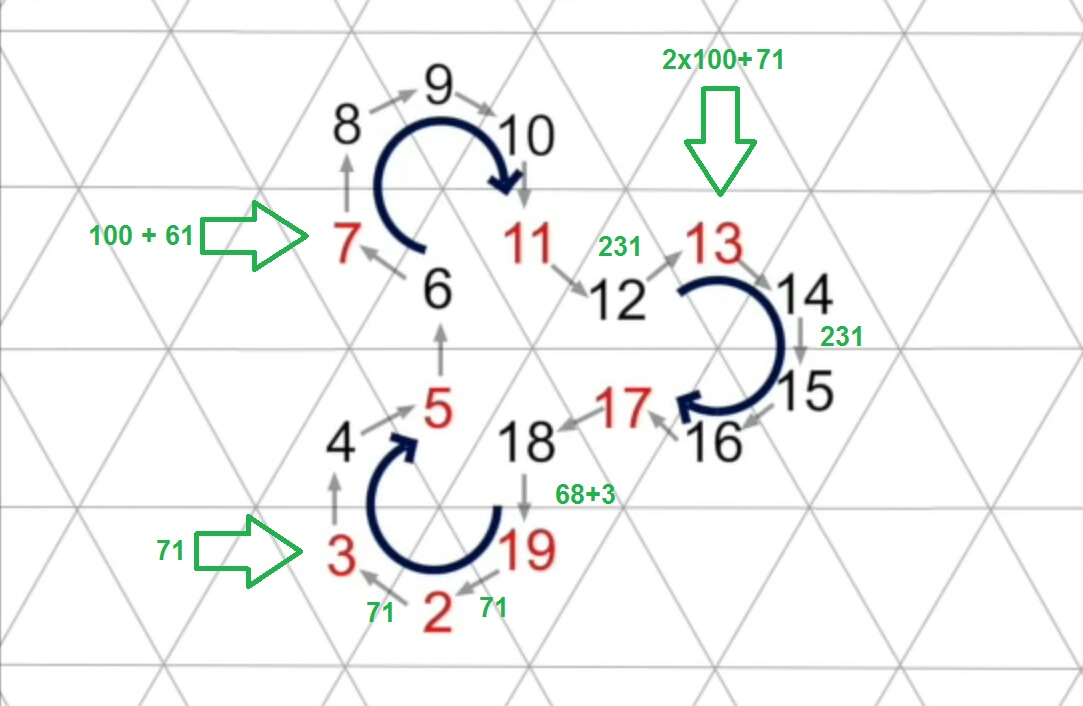This pattern is raised up per six (6) cycles on the 18+1=19, 42+1=43 and 72-1=71. Since the members are limited to the sum of 43+71=114 so here the bilateral way of 19 that originated by the (Δ1) is clearly the one that controls the scheme.

6 x (1 + 6 + (6 + 6)) = 6 + 36 + 72 = 42 + 72 = 43 + 71 = 114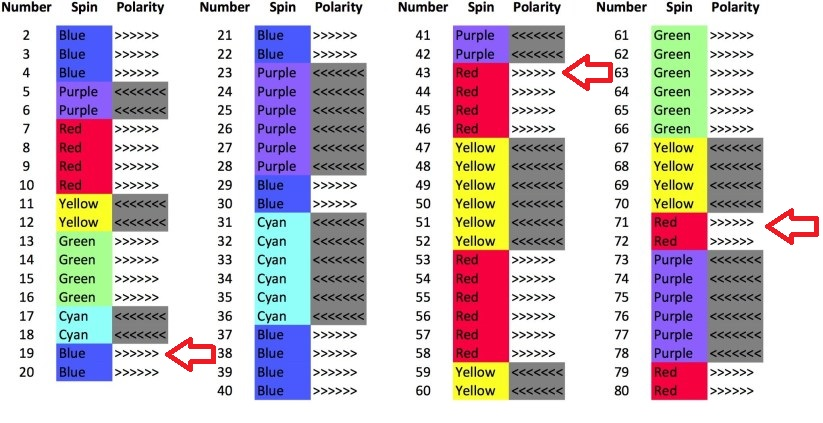This polarity is happened per six (6) cycles by the polar of six (6) to one (1) and six (6) to seven (7) by which we finally found if this behaviour is cascaded bilaterally within the correlation between 61 as the 18th prime and 67 as the 19th prime.

1/7 = 0,142857142857142857142857.. cycling six (6) numbers of (1,4,2,8,5,7) infinity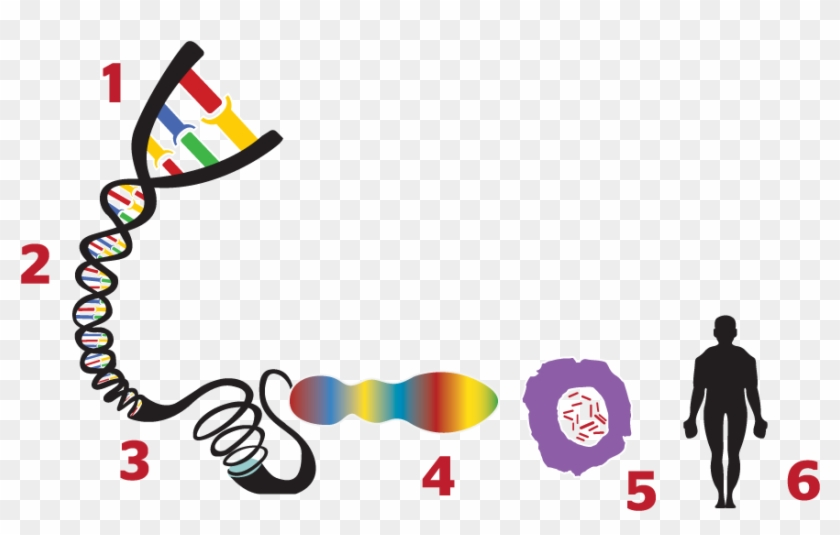This curve is a polar plot of the first 20 non-trivial Riemann zeta function zeros including Gram points along the critical line ζ(1/2+t) for real values of t running from 0 to 50. The consecutive zeros have 50 red plot points between each with zeros identified by magenta concentric rings (scaled to show the relative distance between their values of t).

20x10+ ½(16×6) + ¼(12×18) + ⅛(16×16) = 200 + 48 + 32 + 6 = 286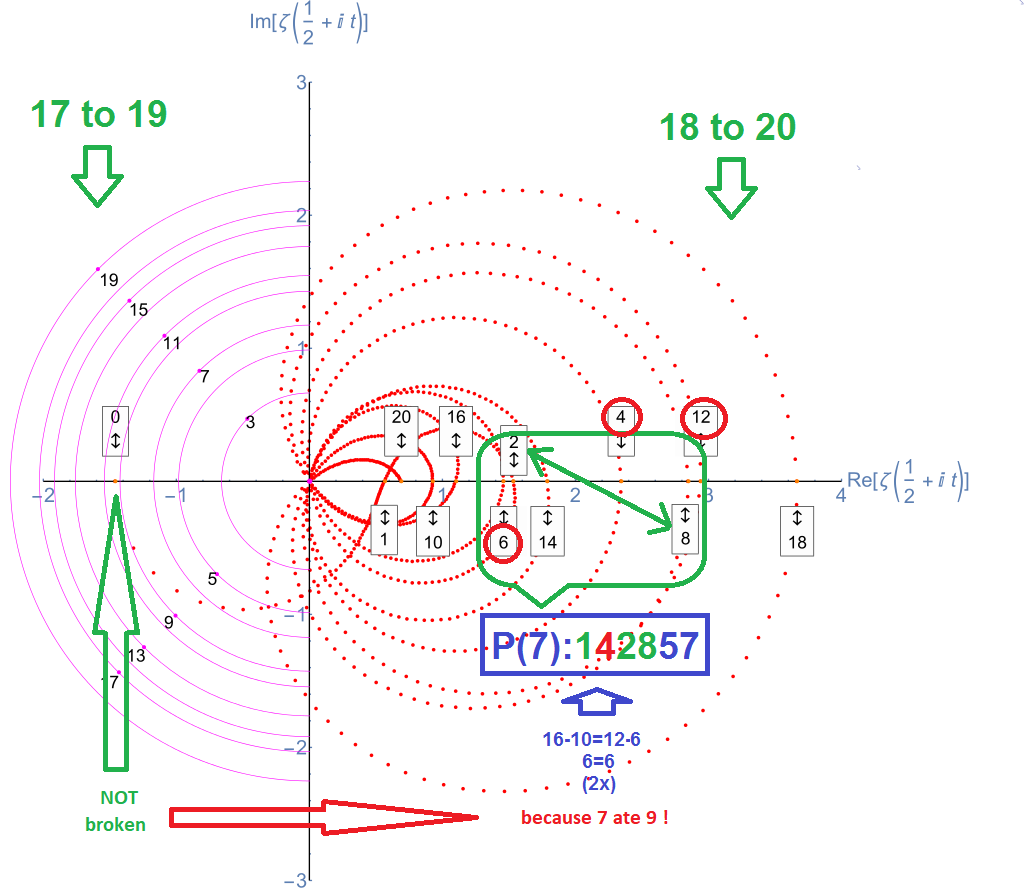See on the left side contains of odd numbers less and equal to 19 while the right is the even number less and equal to 18. By the left side only 9 and 15 that are not primes. Consider these two (2) numbers are laid side by side as part of Fibonacci sequence on the Pascal Triangle. Thus the above curve is actually represesenting the pattern of true-prime-pairs via bilateral 9 sums.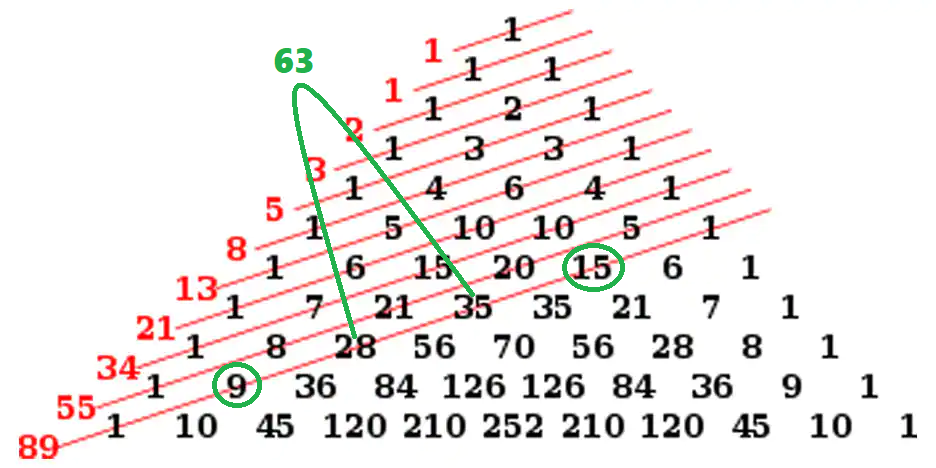Meanwhile the sum of 28 and 35, which is 63, has a digital root of 6+3 = 9 and is landed precisely on the 15's cell of the prime hexagon. So the interaction with the seven (7) objects is made through the object of 36 which has the same digital root of 9.

36® - 9® (1,2,10,47,66,73,86,102,107) - 6°(diagram) - 12® (pinned) = 36® - 27® = 9®As you may see the correlation between each items could involve a complex geometry either by area or volume object that may lead to their tensor and gap. So we decided to assign these behaviours one by one in to a separate group of repositories.

{10,11,12,14,15} & {26,28} = {5®} & {2®} = {7®} = {30,31,32,33,34,35,36}As conclusion to this presentation is that the structure of those prime numbers is not happened by a coincidence. It might hard to believe if they were specifically designed to become the base algorithm on generating the human chromosomes from DNA.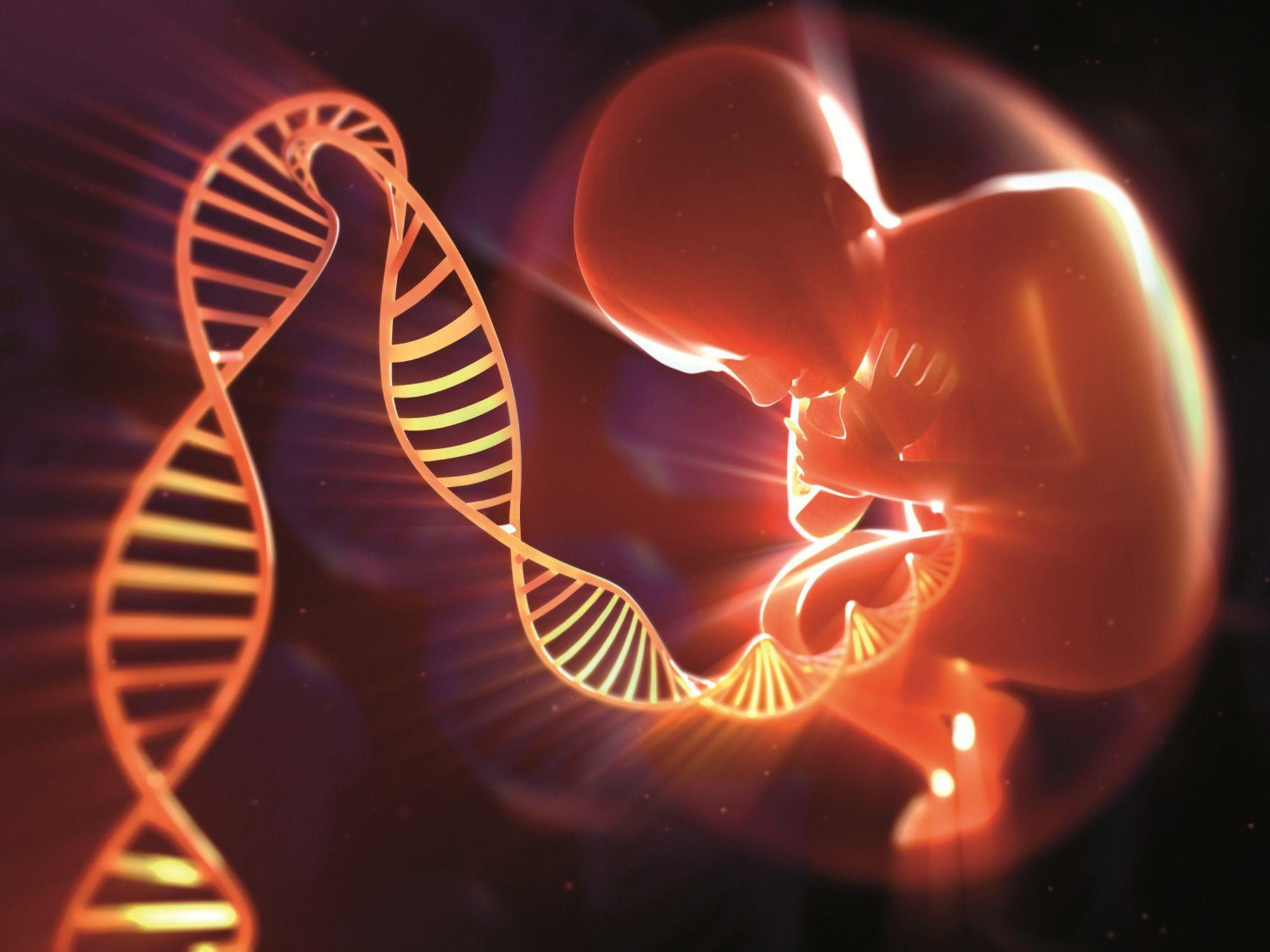By the next parts this will go much more details. Such as the generation of the said DNA from its particular atoms and molecules from the nature of universe which may bring another conclusion if it was initiated by a gap.

₠Quantum Project